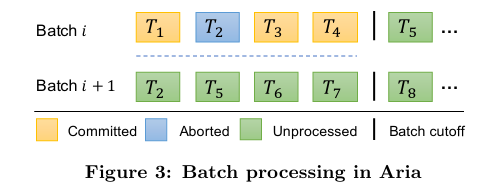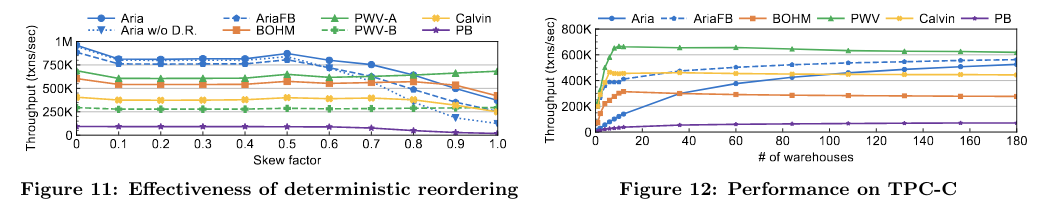## 何为确定性

• 交互式事务会出现死锁状态，出现死锁时，哪个事务被 abort 是不可预知的
• 为了使事务不失败，在有些数据库有 auto retry 的逻辑，这一步也含有不确定性

## 关键技术

### 有序持锁

• 不会出现死锁。假设有两个事务 $ts(T_1) < ts(T_2)$ 如果 $T_1$ 持有 $x$ 上的锁，$T_2$ 持有 $y$ 上的锁，随后在 $T_1$ 尝试去获取 $y$ 上的锁的时候，在交互式数据库中就会出现死锁的现象。而根据有序持锁的要求，此时 $T_1$ 应当持有 $x$ 和 $y$ 上的锁，$T_2$ 则需要等待 $y$ 上的锁释放。
• 不会出现结果不确定的锁竞争。假设有三个事务 $ts(T_1) < ts(T_2) < ts(T_3)$，如果 $T_1$ 持有 $x$ 上的锁，$T_2$ 和 $T_3$ 尝试去获取 $x$ 上的锁，在交互式数据库中他们都会陷入等待的状态，而当 $T_1$ 结束后，$T_2$ 和 $T_3$ 到底谁应该进入到持锁条件中是不确定的。而根据有序持锁的要求，此时应当由更早发生的 $T_2$ 持有 $x$ 上的锁。

Calvin 采用了 Optimistic Lock Location Pre-diction(OLLP)，把事务的运行分为两个阶段，在输入过程中，尝试加锁，输入结束后，再将事务加入到 input log 中，在上面死锁的例子中，$T_1$ 和 $T_2$ 都能够被成功的加入到 input log 中，之后会进入执行阶段，在这一阶段，$T_2$ 会发现自己实际没有持有 $y$ 上的锁（被 $T_1$ 抢占），此时这个事务会 abort，之后被重新加入到 input log 中。

### 事务依赖图

ANSI SQL-92 通过列举 Anomaly 的方式提出了四项隔离级别，也是当今最常用的隔离级别：

• Read uncommitted
• Read committed
• Repeatable reads
• Serializable

「A Critique of ANSI SQL Isolation Levels」这篇文章认为 ANSI SQL-92 的定义过于简略，并对其做了补充，提出了一些新的 Anomaly，其中的 Snapshot Isolation 因为和 MVCC 的吻合度高，被被广泛使用。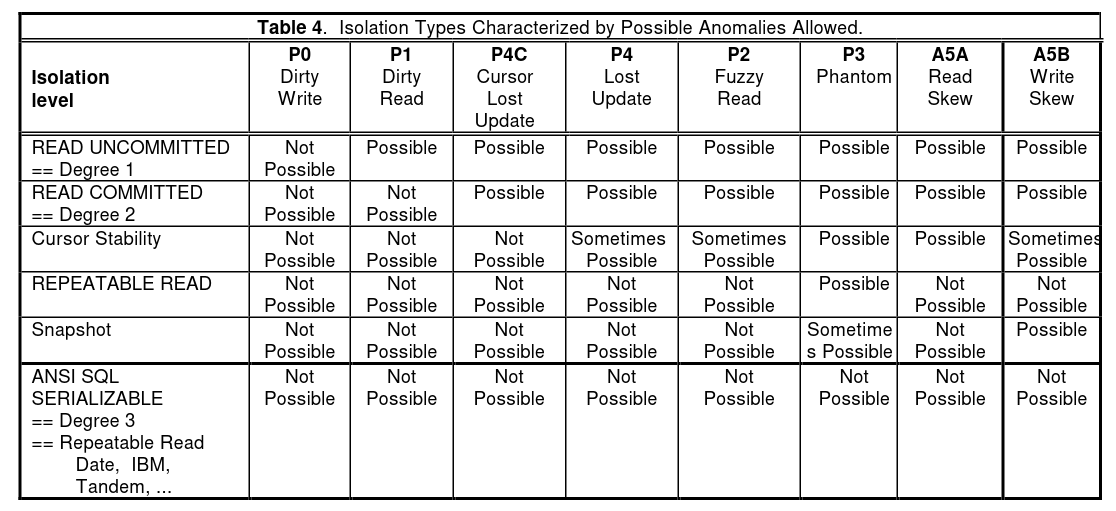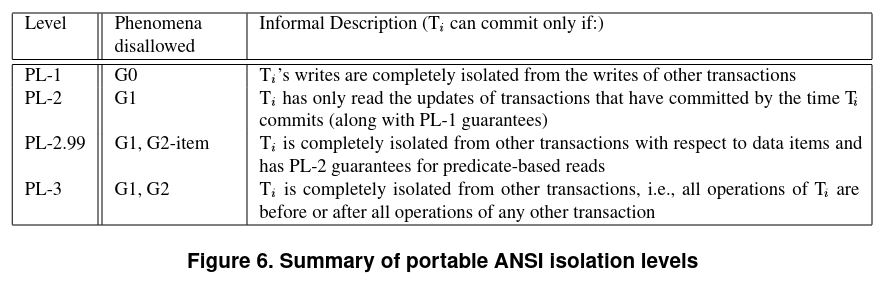$T_1$ $T_2$ $T_3$
$w(x, 1)$
$w(x, 2)$
$r(x)$

### Coordination

The only coordination that needs to happen in a deterministic database system is the communication required to agree on the input to the system.

Deterministic Commit Two-phase commit
Coordination the input log(ordering) Prewrite with lock
Commit input log Commit & erase lock | primary
Commit & erase lock | secondaries

2pc 的一大优势是水平扩展容易，因为它在执行时候不需要考虑事务与事务之间的关系，而 Deterministic 的 ordering 步骤在大规模并行化的事务提交场景中，成本也会随之升高，尤其是分布式 ordering，至今仍是一大难题。

## 历史上的确定性数据库

### H-Store

• Single-sited Transactions，只存在于一个 site 上的事务，将事务发送到对应的 site 上去执行
• One-shot Transactions，涉及到多个 sites，但是每一条语句只存在于一个 site 上

### Calvin

「The Case for Determinism in Database Systems」设计了一个接受所有事务请求的节点，这个节点还会将事务持久化，然后按照接收到事务的 ts 将事务加入到 input log 里面去。这个设计存在的问题在于单点，包括出现故障时无法恢复和扩展规模时候的单点性能瓶颈。Calvin 添加了一个 sequencer layer。scheduler1 scheduler2 scheduler3
$T_1(w(a, 1))$
$T_2(w(a, 2))$ $T_2(w(b, 2))$ $T_2(w(c, 2))$
$T_3(w(c, 3))$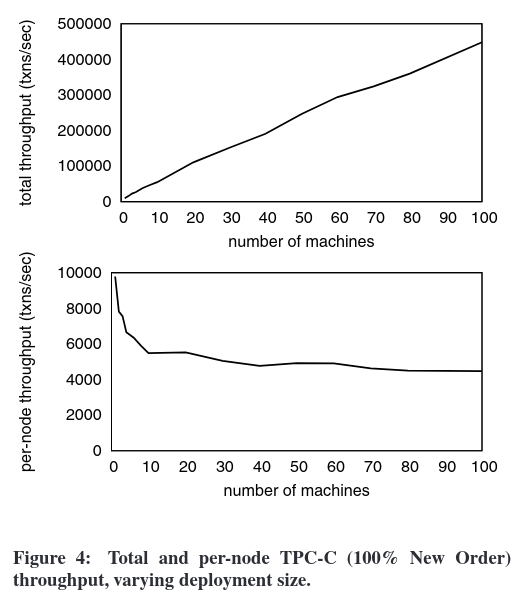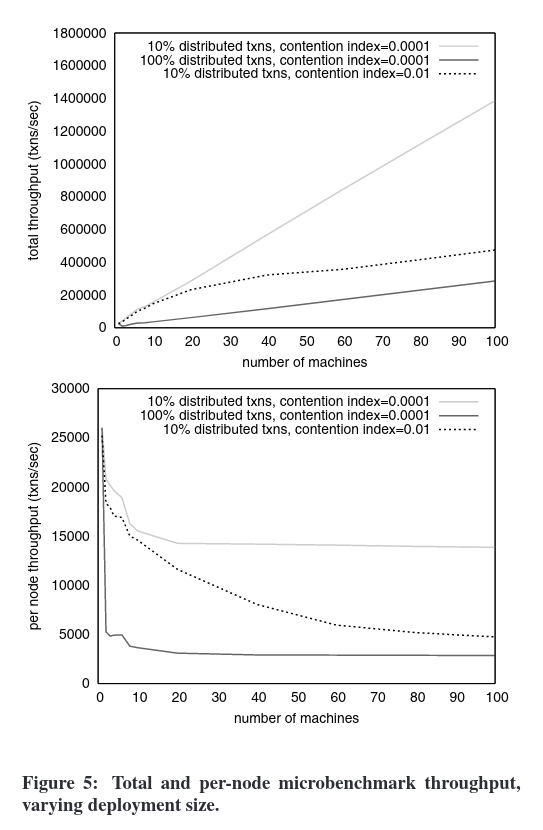### BOHM

BOHM 的特点在于：

• 中心化递增的 ts 分配
• 改变了 MVCC 的实现方式，让 Serializable 的实现不以大量牺牲性能为代价
• 引入了事务依赖图，让原本不能并发执行的事务变得可并发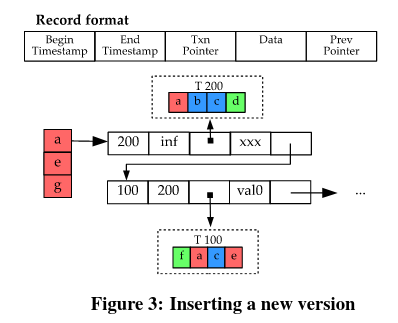BOHM 的 version 有五个字段

• Begin ts，数据写入的 ts
• End ts，数据失效的 ts
• Txn pointer，写入该 version 的事务指针
• Data
• Prev pointer，上一个版本的指针

Version 的有效期是 。图中 $T_{100}$ 和 $T_{200}$ 这两个事务都尝试写了 $a$ 这个 key，于是创造了两个版本，一个 version 的有效期是 ，另一个是 。Txn pointer 指针分别指向写入他们的事务，Prev pointer 指针指向前一个 version， $T_{200}$ 对应的 version 的 Data 字段为为未初始化。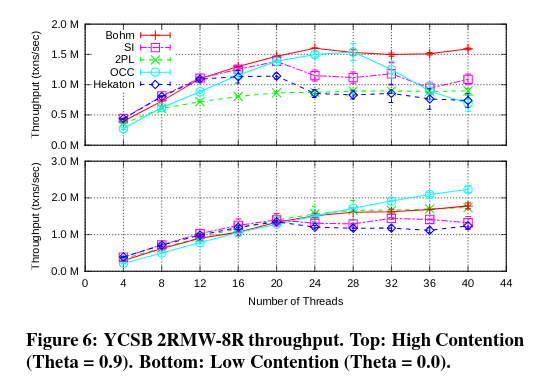Benchmark 表明，越高冲突的场景，BOHM 的效果越好，在高冲突高并发的情况下，乐观事务模型出现了大幅度的性能回退。

### PWV

• 提交可见性，一但一个事务完成了提交，它所写入的数据就能够被其他事务所读取到，其优点在于事务与事务的耦合性低。但是在一个 Serializable 的系统中，这一策略可能会增加延迟、降低整体的并行度。
• 写入可见性，一个事务即使没有提交，他所写入的数据就可以被其他事务读到，但是如果这个事务最后失败了，读取到其数据的事务也需要 abort 防止脏读。Abort 是连锁式的，可能会付出极大的代价。

• 逻辑原因，事务违反了约束条件
• 系统原因，包括死锁，系统错误，乐观事务中的写冲突

• 逻辑原因，同上
• 写入可见带来的 abort，$T_2$ 需要根据 $T_1$ 的写入值去修改某个值，这一修改可能会因 $T_1$ 的结果产生逻辑原因的 abort

• 在 commit point 之前的，可能会 abort，需要在 commit point 之后被读取到
• 在 commit point 之后的，不会 abort，一但写入成功就能被读取到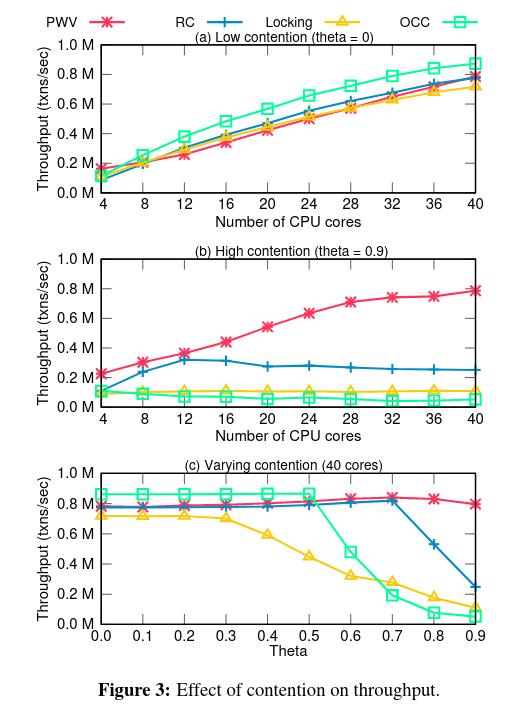PWV 的论文实现还有许多细节，核心思想就是让事务在完全提交前就能被读取到的同时处理写写依赖，其实现远比想法复杂，这里不再详细展开了。

### Aria

BOHM, PWV 和 Calvin 的策略都是定序-执行两阶段，Aria 提出了一个新的想法，如果定序和执行能够放在一起，是否能取得更好的性能、以及在不同场景下更加均衡的表现（更好的泛用性）呢？

Aria 在执行阶段采用 reservation 的机制，并且以全局递增的事务 id 作为优先级的标准（这一机制是达成 deterministic）的关键。如果 $ts(T_1) < ts(T_2)$，$T_2$ 对 $x$ 做了 reservation，当 $T_1$ 也尝试对 $x$ 做 reservation 的时候，会发现 $x$ 被一个 id(此处为 ts) 比它大的事务占有了，于是就会发生强占。